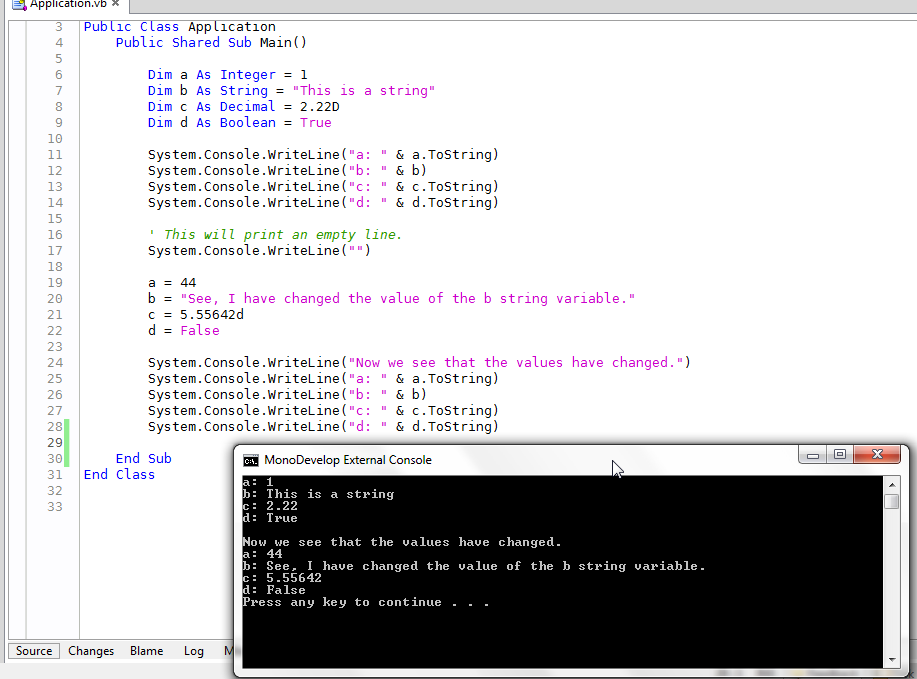# Variables - Basic

Variables are the basic working blocks in code. You use variables to hold values. There are several different variable types but in this lesson we will cover only four of them.

To see the example code that goes along with lesson go to https://github.com/majorsilence/VB-Notes/blob/master/VbBook1/BasicVariables/Application.vb.

To declare a variable you use the language keyword “Dim” used with a name and “As”. So if you want a string called “Hello World” named TestVariable you would declare it like this.

``````Dim TestVariable As String = "Hello World"
``````

This example declares a variable and assigns a value at the same time. However you can declare a variable without assigning value. The value can always be assigned later. A good general rule is only declare a variable when it is ready to be used (assigned) when possible.

• Integer - are like whole numbers but can contain negatives
• String - contain multiple characters
• Decimal - numbers with decimals
• Boolean - is true or false

# Integers

Integers are like whole numbers but can contain negatives. So they have negatives, zero, or positives.

For example -5, -4, -3, -2, -1, 0, 1, 2, 3, 4, 5 are all integer values.

To declare an integer you can do this.

``````Dim i As Integer
``````

This example creates a new variable of type Integer name i. It does not assign any value to i. To assign a value to i you can do it like this.

``````i=1
``````

Now the value of i is 1.

``````i=2
``````

Now the value of i is 2.

# Strings

Strings can hold any value. They can have letters, numbers, special characters. They can be long or short

To declare a string you can do this.

``````Dim s As String
``````

This example creates an empty string called s. This string has no value

To assign the value “hello world” to the variable s we would do.

``````s = "hello world"
``````

The value of the variable can be reassigned at any time so if we want to change the value to “purple monkey dishwasher” just do the same as above put with the new string.

``````s = "purple monkey dishwasher"
``````

If we want to see the value of a variable printed to the console we can write.

``````System.Console.WriteLine("The value of s is: " & s)
``````

We see here that s is value is append to the string “The value of s is: “ and then printed to the console as “The value of s is: purple monkey dishwasher”. You can append any string to any other string at any time using the & symbol.

## StringBuilder

If you are appending to a string again and again or changing it value over and over again this can become very slow. String operations like this can be speed up using the StringBuilder class. I will not go into detail here but you can read more at http://blogs.msdn.com/b/irenak/archive/2005/11/28/497420.aspx.

To use a string builder you need to initialize System.Text.StringBuilder.

``````Dim builder As New System.Text.StringBuilder
builder.Append("Hello Word ")
builder.Append("Peter.  ")
builder.Append("Have a good day.")

System.Console.WriteLine(builder.ToString)
``````

This will print “Hello World Peter. Have a good day.”.

What this does is keep adding to a buffer and when you call the ToString method it finally creates a string. This is much faster then concatenating the string together like the following.

``````System.Console.WriteLine("Hello World " & "Peter.  " & "Have a good day.")
``````

This example probably is not faster since it is so tiny but if you did this with 100 000 strings the string builder would be much faster.

# Decimals

Decimals are used when you need numeric values that contain decimals places. It is essential if you are doing financial calculations that you use decimals and no other data type. See the section Doubles are Bad - Financial Calculations for more details.

For example -5.32, -4.76, -3.7654, -2.1, -1.343, 0.13, 1.786555, 2.2, 3.765, 4.22, 5.3446 are all decimal values.

To declare a decimal you can do this.

``````Dim d As Decimal
``````

This example creates a new variable of type Decimal with the name d. It does not assign any value to d. To assign a value to d you can do it like this.

``````d=5.437D
``````

Now the value of d is 5.437.

``````d=2.55
``````

Now the value of d is 2.55. As shown above variables in function can always be reassigned new values

# Booleans

Booleans are variables that can be either True or False. That is all they hold.

To declare a boolean you can do this.

``````Dim b As Boolean
``````

This example creates a new variable of type Boolean named b. It does not assign any value to b. To assign a value to b you can do it like this.

``````b=True
``````

Now the value of b is True.

``````b=False
``````

Now the value of b is False.

There is no other value that a boolean can hold. If you do not set a value a boolean will default to False.

# Screenshot

This screenshot shows the output of the example application when it is run.